# Calculating Linear Actuator Force for Your ApplicationOrlaith Morris
PA Engineer

This article is dedicated to walking you through the process of calculating the force of an actuator required to successfully lift the load of your application. There are a couple of factors involved to find the right force, including the load, the angle at which it is being pushed and the size of the load.

Most of our actuators have customizable force!

## Calculating the Required Force of a Linear Actuator

There are a variety of terms and variables that you would need to understand before attempting to determine the force on a linear actuator. They are as follows.### Linear Actuator Force Calculation Process

This sample calculation will show you how to calculate the force of a linear actuator when it needs to thrust 200 lbs of mass a full 8” within a time limit of two seconds. You would need to work from the assumption that the load which needs to be moved is inclined at thirty degrees, meaning that the leaning coefficient of friction will be 0.15. While doing this linear actuator force calculation, you should also work from the assumption that there is an opposing force of twenty-five pounds working against the actuator.

Calculating total force is the first step in the equation for determining which size of linear actuator would be best. This process considers four elements to come up with a proper answer, namely friction, acceleration, gravity, and applied force. The actual equation to calculate linear actuator force is as follows.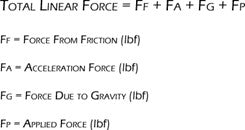When this equation has been done, the next area to move onto is calculating the total force equation. This would be done using the equation below.Finally, the last phase of the equation is using the numbers previously supplied.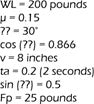These would then be used in the final equation which should take on a form similar to the one below.

The actual calculation gives us an answer to the question about linear actuator force, which is: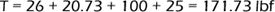Choose the right control system for your actuator and take a look through our range!

## Straight up Versus Angled Mount

The direction of linear actuator force determines the calculation that needs to be used. The actuator can be mounted straight up or at an angle. Below, we discuss both configurations and how to calculate the force of the linear actuator in each scenario.

### Mounted Straight Up

To calculate an actuator’s push-pull force if it is mounted straight up, the actuator required should be the same force rating as your required weight. However, one should always opt for an actuator with a higher force than the load to be on the safe side. This is due to friction and other factors which can increase the force required to move the load. As in the picture below, for example, if the load is 50 lbs then the force rating (F) of the actuator should be greater than 50 lbs.## Mounted at an Angle

When the load and the actuator need to be mounted at an angle, the means to calculate actuator force changes quite significantly. As the actuator will be pushing at an angle, in most cases it will need a higher force than the actual load in order to lift it.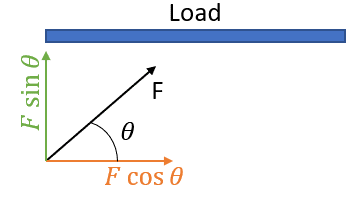The above figure perfectly demonstrates the factors one must consider when calculating force for an angled mount. For example, if the load is 50 lbs and it is mounted at a 45° angle (θ) to the ground, one must ensure that the force perpendicular to the load, F sinθ, in this case, is greater than or equal to 50 lbs.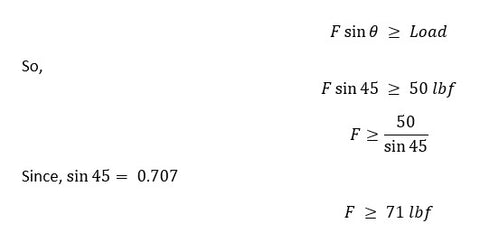This means that if you are pushing a load of 50 lbs at a 45° angle, you would need a linear actuator force that can handle pushing at least 71 lbs.

Alternatively, if you are planning to push the load sideways and would like to push it at an angle of 60°, it is the same equation but altered to account for the direction you are pushing your application’s load. This is shown in the image below.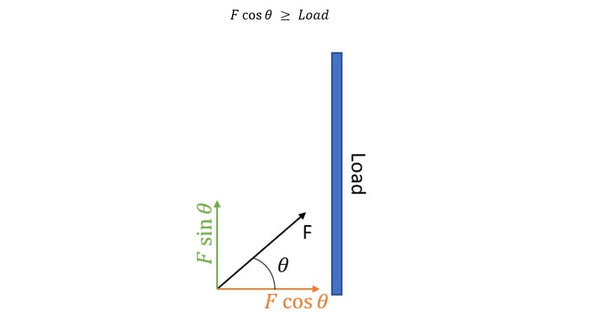You will also be using the same method as shown previously, as per the below equation.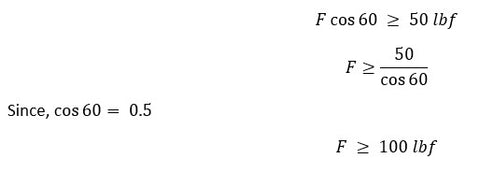Use our Actuator Compare Tool to check and compare multiple actuators at once!

## Converting Linear Actuator Force from Pounds to Force into Newtons

In case you need to convert the result of the calculation from pound-force (lbf) to Newton (N), remember that one pound-force equals 4.45 Newtons. It means one lbf = 4.45 N. From the example above, for example, had the result of 171.73 lbf. Thus, in newtons, it will be 764 N (171.73 * 4.45).

Final Word

To sum up, the equations mentioned in this article represent each scenario whereby your linear actuator’s mounting is perpendicular to the load. Use these calculations to determine the required force rating of your actuator, ensuring you are never undercutting the amount you will need. It is a simple step but can help save you a lot of time when selecting the right linear actuator force. Progressive Automations provide multiple guides and resources to select the right linear actuator for your application. Once you have selected the right force, you can determine the stroke, voltage, and what control methods you would like to use. If you do require further assistance on how to choose a linear actuator for your application, give us a call at 1-800-676-6123, or email us at sales@progressiveautomations.com.